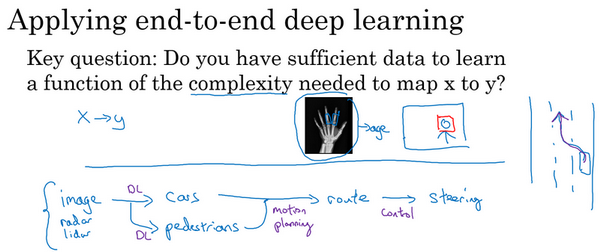## 第二周：机器学习策略（2）(ML Strategy (2))

### 2.1 进行误差分析（Carrying out error analysis）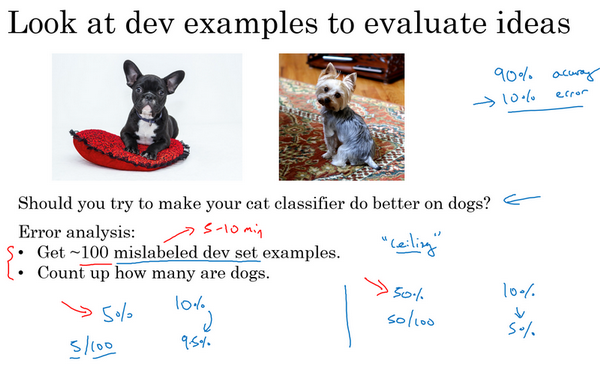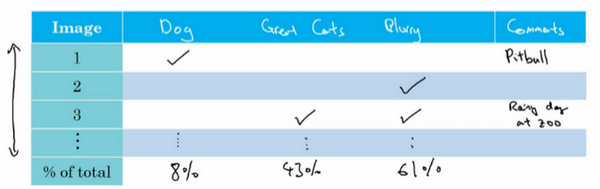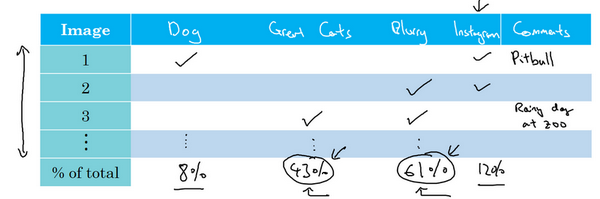### 2.2 清除标注错误的数据（Cleaning up Incorrectly labeled data）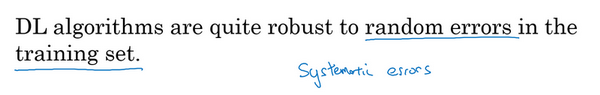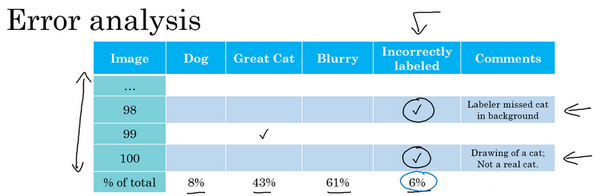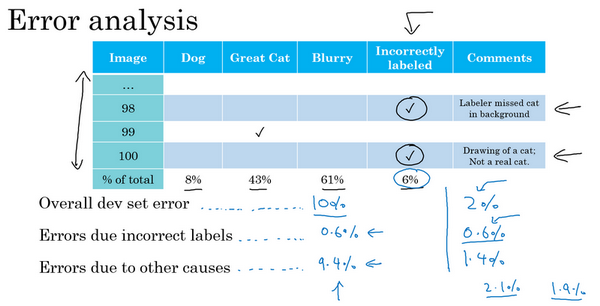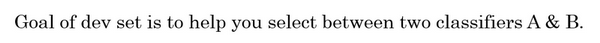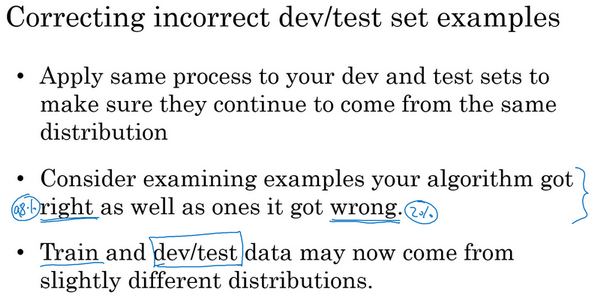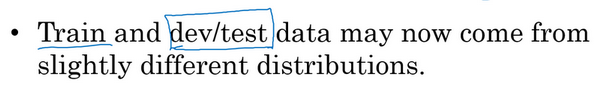### 2.3 快速搭建你的第一个系统，并进行迭代（Build your first system quickly, then iterate）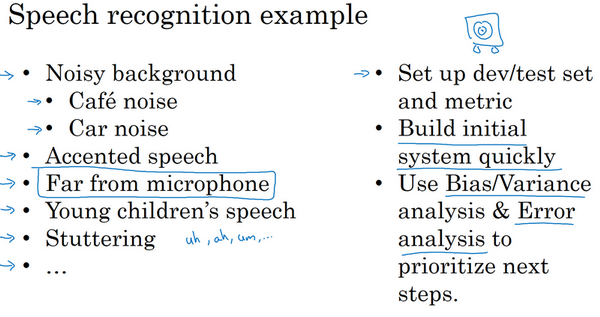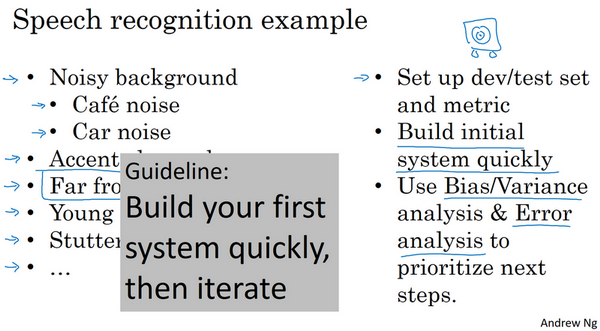### 2.4 使用来自不同分布的数据，进行训练和测试（Training and testing on different distributions）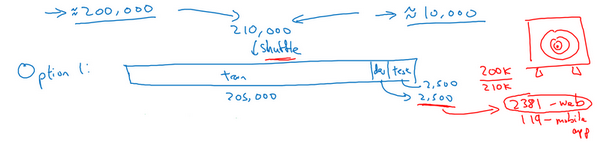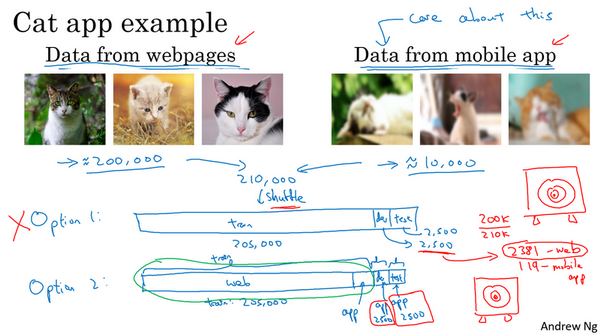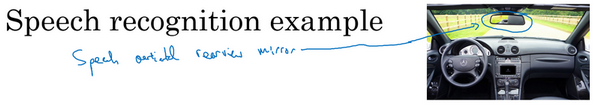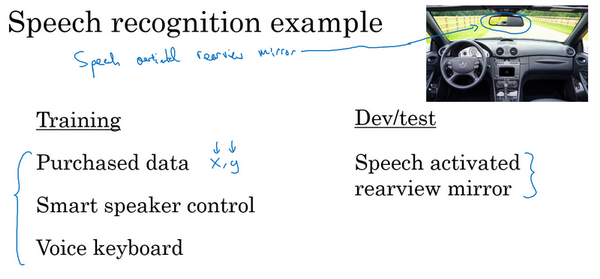### 2.5 数据分布不匹配时，偏差与方差的分析（Bias and Variance with mismatched data distributions）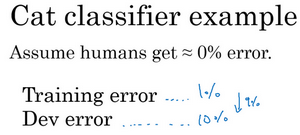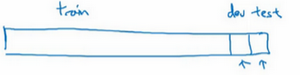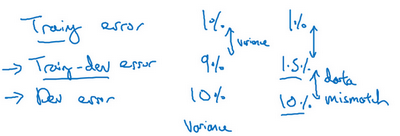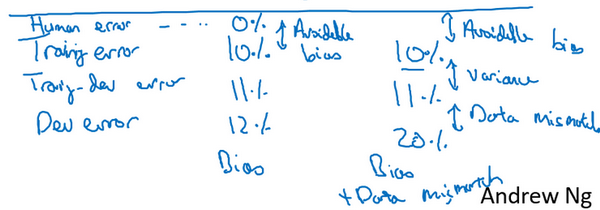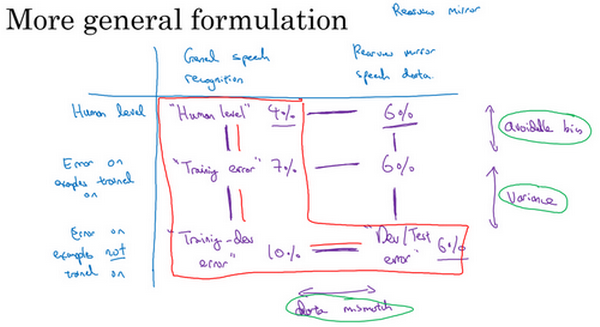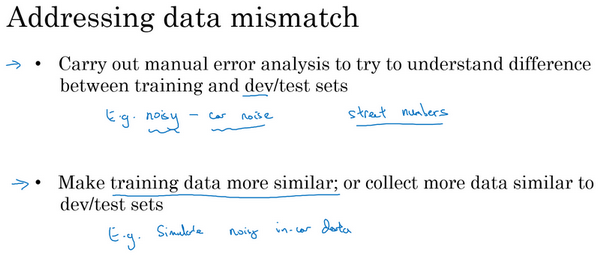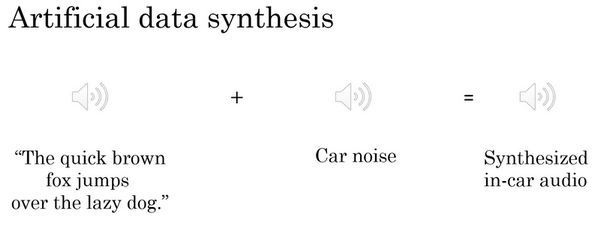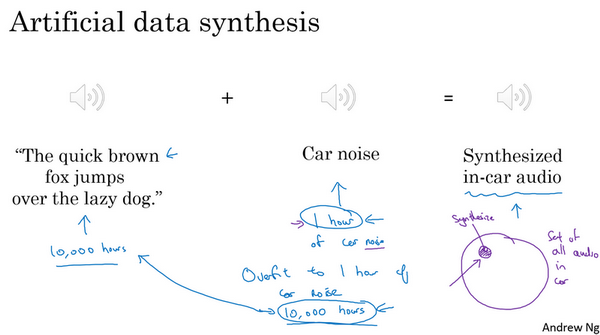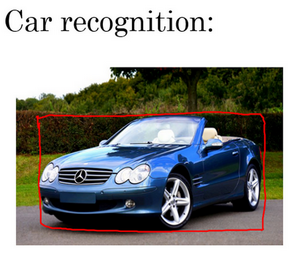### 2.7 迁移学习（Transfer learning）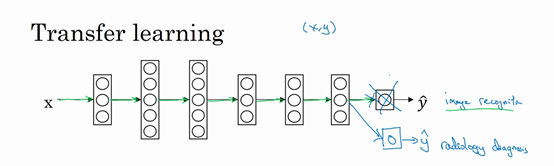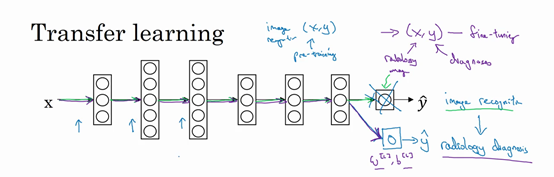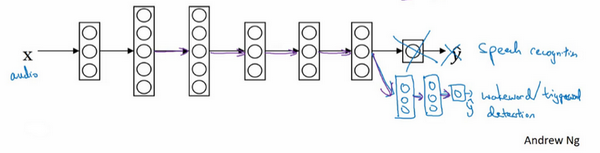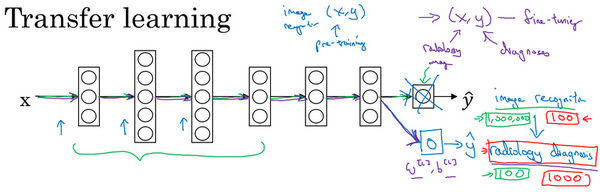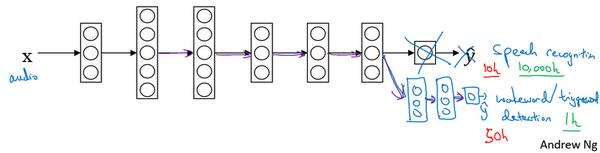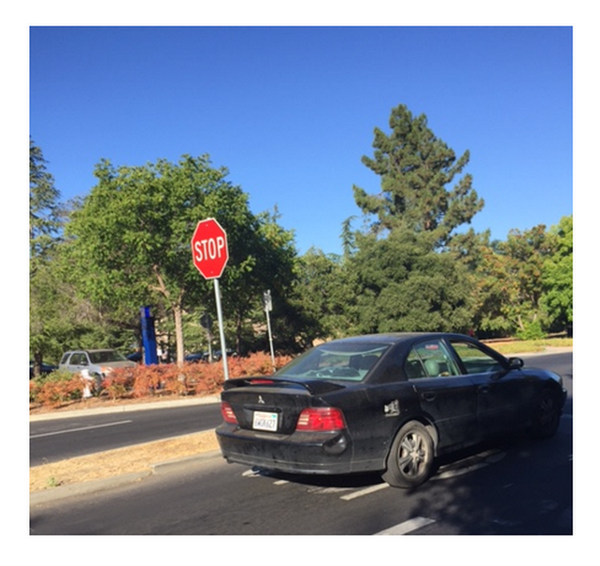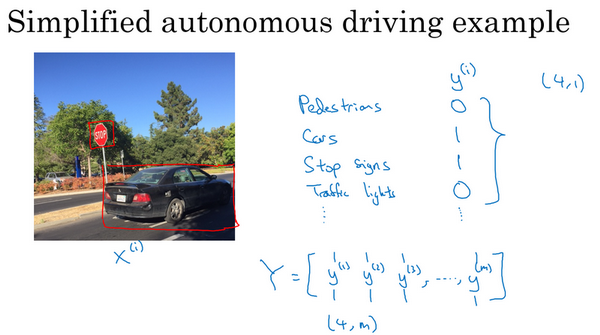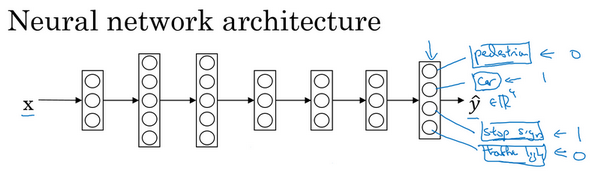$\frac{1}{m}\sum_{i = 1}^{m}{\sum_{j = 1}^{4}{L(\hat y_{j}^{(i)},y_{j}^{(i)})}}$

$\sum_{j = 1}^{4}{L(\hat y_{j}^{(i)},y_{j}^{(i)})}$这些单个预测的损失，所以这就是对四个分量的求和，行人、车、停车标志、交通灯，而这个标志L指的是logistic损失，我们就这么写：

$L(\hat y_{j}^{(i)},y_{j}^{(i)}) = - y_{j}^{(i)}\log\hat y_{j}^{(i)} - (1 - y_{j}^{(i)})log(1 - \hat y_{j}^{(i)})$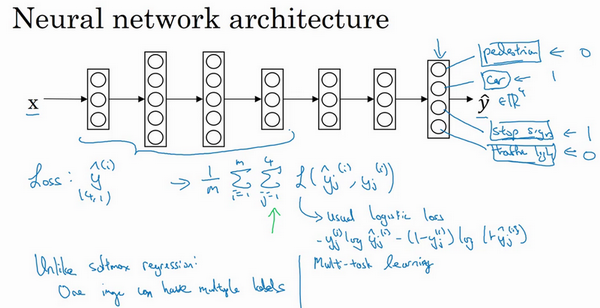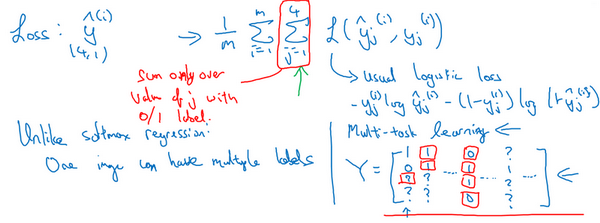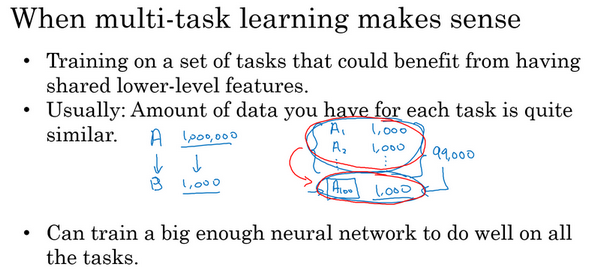### 2.9 什么是端到端的深度学习？（What is end-to-end deep learning?）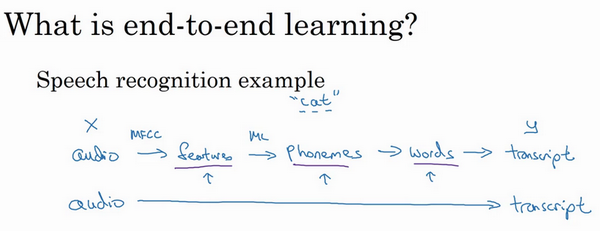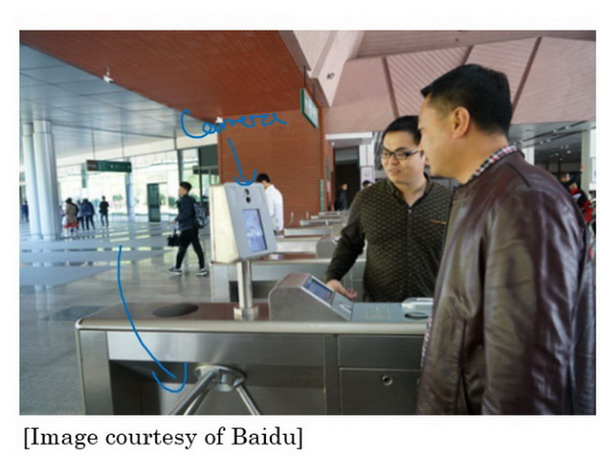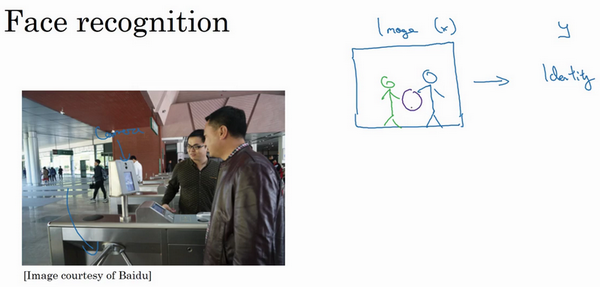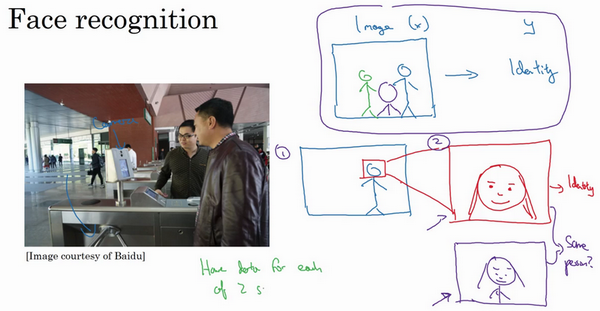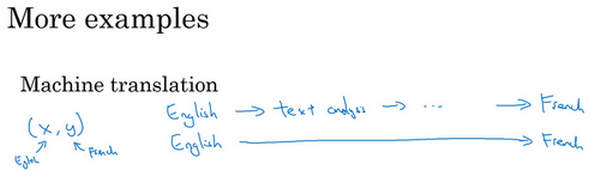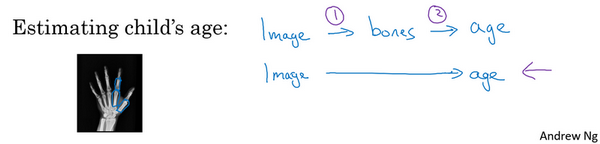### 2.10 是否要使用端到端的深度学习？（Whether to use end-to-end learning?）